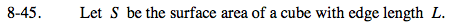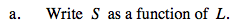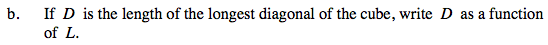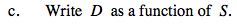### Home > PC > Chapter 8 > Lesson 8.1.3 > Problem8-45

8-45.S = 6L2Draw a triangle within the cube with the longest diagonal D.

Find the diagonal of the bottom square AC.

$\overline{AC}=\sqrt{L^2+L^2}$

$\overline{AC}^2=L^2+L^2$

$\overline{\textit{AC}}=\sqrt{2\textit{L}^2}$

$\overline{\textit{AC}}=\textit{L}\sqrt{2}$

$\text{Find longest diagonal }, D.$

$D^2=L^2+(L\sqrt2)^2$

$D^2=L^2+(2L^2)$

$D^2=3L^2$

$D=L\sqrt3$Solve for L in your equation from part (a) above. Then substitute that expression for L in your D equation.

Use the eTool below to solve this problem.
Click the link at right for the full version of the eTool: PCT 8-45 HW eTool